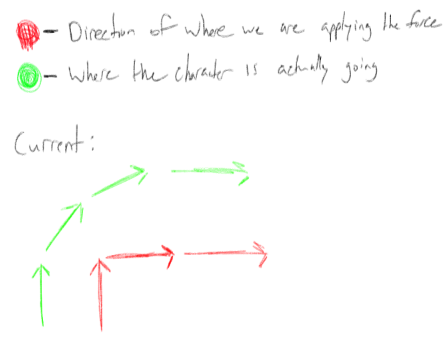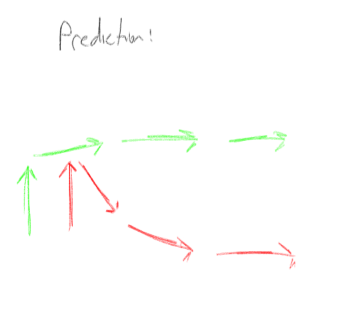# Custom Movement Help (Vector Force)

Hello! I’m currently trying to create a custom movement system similar to this game on Roblox Studio!

I suspect they used vector force to create their movement system, so I did just that.

After disabling the default controls, animations, and making the character into physics mode. I applied anti-gravity and aligned orientation, to reduce friction and keep the player standing.

I successfully made it so that when the player pressed the WASD keys the player can move around.
A vector force called “MovementForce” is added to the HumanoidRootPart and goes in the direction corresponding to which the key is pressed

I also made it so that when the player goes too fast, another Vector force object tries to cancel the momentum by applying a force in the opposite direction. A Vector force called “CancelForce” is added to the HumanoidRootPart, and a function is binded to the renderstep, that checks and sets the force.

The problem I am having is that when the player moves forward, then left, an arc/curve is formed. Which slowly moves the player in the requested direction. As shown here:

However in Ragdoll Universe’s Movement System the turn is more immediate with minimal arc/curve:

How would I go about achieving this goal?

I have tried adding a “HelperForce” which applies a force in the direction you pressed your key in, enabling and disabling after a short period of time, to help set the character on the right track. Sadly this does not work.

Currently, I am experimenting with applying a cancel force every time the player releases a key.

Any ideas? :DD

Code:

``````

local BaseMovement = {}
BaseMovement.__index = BaseMovement

--- Services
local ContextActionService = game:GetService("ContextActionService")
local Players = game:GetService("Players")
local RunService = game:GetService("RunService")

local LocalPlayer = Players.LocalPlayer

--- Modules
local KeyBinds = require(script.Parent.Parent.Keybinds)

--- Local Func

function BaseMovement.New(value)
local self = setmetatable({}, BaseMovement)

self.SPEED = 2000
self.CANCEL_SEVERITY = 1

self.GRAVITY_LEVEL = 0.5
self.MOMENTUM_CAP = 40
self.JUMP_HEIGHT = 20000

self.onGround = false
self.justLanded = false
self.isMoving = false

-- may not need these
self.GravityForce = false
self.MovementForce = false

return self
end

function BaseMovement:Enable()

self:Disable()

self:SetForces()    -- puts all neccesarry forces into the character
self:SetAlignment() -- puts all neccesarry alignment into character

local inputFunctions = {
[KeyBinds.keyboardWalkForward] = function(actionName, inputState, inputObject)
if inputState == Enum.UserInputState.Begin then
--print("Pressed 'W'")
self.MovementForce.Force += Vector3.new(0,0,-self.SPEED)
else
--print("Released 'W'")
self.MovementForce.Force += Vector3.new(0,0,self.SPEED)

end

--print(self.MovementForce.Force)
end,
[KeyBinds.keyboardWalkLeft] = function(actionName, inputState, inputObject)
if inputState == Enum.UserInputState.Begin then
self.MovementForce.Force += Vector3.new(-self.SPEED,0,0)
--print("Pressed 'A'")
else
self.MovementForce.Force += Vector3.new(self.SPEED,0,0)
--print("Released 'A'")
end
end,
[KeyBinds.keyboardWalkBackward] = function(actionName, inputState, inputObject)
if inputState == Enum.UserInputState.Begin then
self.MovementForce.Force += Vector3.new(0,0,self.SPEED)
else
self.MovementForce.Force += Vector3.new(0,0,-self.SPEED)
end
end,
[KeyBinds.keyboardWalkRight] = function(actionName, inputState, inputObject)
if inputState == Enum.UserInputState.Begin then
self.MovementForce.Force += Vector3.new(self.SPEED,0,0)
else
self.MovementForce.Force += Vector3.new(-self.SPEED,0,0)
end
end,
[KeyBinds.keyboardJump] = function(actionName, inputState, inputObject)
print("Space was pressed")
end,
}

local inputs = {}

for input, func in pairs(inputFunctions) do
table.insert(inputs, input)
end

ContextActionService:BindAction("Movement", function(actionName, inputState, inputObject) -- this method may not be useful for movie buttons when setting positions, useful only when it is "False"
if inputFunctions[inputObject.KeyCode] then
inputFunctions[inputObject.KeyCode](actionName, inputState, inputObject)
elseif inputFunctions[inputObject.UserInputType] then
inputFunctions[inputObject.UserInputType](actionName, inputState, inputObject)
end
end, false, table.unpack(inputs))

RunService:BindToRenderStep("temp", Enum.RenderPriority.Camera.Value, function()
--
--[[]]
local velocity, magnitude = self:GetPlayerAxisMagnitude(true,false,true)

local desiredDirection = self:GetPlayerMovementVector3(true,false,true).Unit
if self.MovementForce.Force == Vector3.new(0,0,0) then
desiredDirection = Vector3.new(0,0,0)
end

if magnitude > self.MOMENTUM_CAP then -- need to compare and check directions using vector.unit
--apply cancel

self.CancelForce.Force = self:GetPlayerMovementVector3(true,false,true) * -1 * self.CANCEL_SEVERITY
self.CancelForce.Enabled = true
else
-- disable cancel

self.CancelForce.Force = Vector3.new(0,0,0)
self.CancelForce.Enabled = false
end

end)

end

function BaseMovement:Disable()
--print("Disabled")
ContextActionService:UnbindAction("Movement")
RunService:UnbindFromRenderStep("temp")
end

function BaseMovement:GetMass(Model)

local mass = 0

for i, v in pairs(Model:GetChildren()) do

if v:IsA("BasePart") then

mass+= v:GetMass()
end
end

return mass
end

function BaseMovement:GetPlayerAxisMagnitude (x,y,z)
local velocity= LocalPlayer.Character.HumanoidRootPart.Velocity

if x == false then
velocity -= Vector3.new(velocity.X,0,0)
end
if y == false then
velocity -= Vector3.new(0,velocity.Y,0)
end
if z == false then
velocity -= Vector3.new(0,0,velocity.Z)
end

return velocity, velocity.Magnitude
end

function BaseMovement:GetPlayerMovementVector3(x,y,z)
local vector = self.MovementForce.Force

if x == false then
vector -= Vector3.new(vector.X,0,0)
end
if y == false then
vector -= Vector3.new(0,vector.Y,0)
end
if z == false then
vector -=Vector3.new(0,0,vector.Z)
end
return vector
end

function BaseMovement:PrintTable()
print("BASE_MOVEMENT:")
print(self)
print(getmetatable(self))

self:PrintTable2()
end

return BaseMovement
``````
2 Likes

[dont mind this]

1 Like

edited the post
thanks for helping!! :DD

You there bro? 0_0
I put the code on the post
:(((

I noticed the cancel force is only a flat amount.

Have you tried a drag force which scales based on the players speed?

1 Like

TyI’ll try this when I get the chance

uhhhhh, you keep deleting your post before I have the chance to respond lol,

Anyways, What I wanted to say was your drag force equation is a lot better than mine, so thanks for providing me with that :DD

and you said that ragdoll universe’s doesn’t change states for its movement? I’m pretty sure that vector force can’t be used anymore if that’s the case…

1 Like

I am deleting them since I think they were incorrect ( just a little ). But yes, that does seem to work if you set the HumanoidRootPart’s density to something lower than 0.7.

1 Like

kk I’ll try this when I have the time

Just tried it, and it didn’t work, I’ve set the HumanoidRootparts density to 0.3 and I walk really slowly…

Reset the `AssemblyLinearVelocity` when the input is changed AssemblyLinearVelocity

Didn’t work, this makes the movement snappy and makes the player stop for a moment. I only applied it to the “W” key in the video, but as you can see I don’t think this what ragdoll universe used:

So this is what I have in mind so far,

Right now how we change directions by having a vector force object in the humanoidrootpart, and changing it’s force value, whenever we press a key. Pressing forward will change the force to Vector3.new(0,0,1) and pressing left will change the force to Vector3.new(-1,0,0)

As shown in the picture I drew, using this method will create a curved path. The green arrows represent the direction the character is going, and the red arrows are the direction of the force that we are applying.To counter this, what if we made the force we’re applying also a curve?If we overshot the direction of the force we’re applying and create a counter curve, I believe we can cancel out the character’s arc.

Do you guys think this will work? If so, how exactly will we be able to change the vector force so that it does what we want?

I believe in Ragdoll Universe they are using BodyVelocity. LinearVelocity should work now that BodyVelocity is deprecated.

I think you are experiencing a curve because you are applying a force in one direction and then applying a force in a different direction. Say you apply a force in the north direction and have 0 friction and then apply the force in the east direction, your character should then head northeast. If you want snappier controls then I would assume that you need to apply more friction. Either increase the friction via the part attribute or through a script.

I’m currently trying to work this out myself.

There’s a really helpful description of a similar thing here Making A Physics Based Character Controller In Unity (for Very Very Valet) - YouTube

It’s for Unity, but the concepts should be universal. If you want to work on this together let me know I’ll keep you updated.

Edit… Roblox staff are currently working on a physics based character controller too.

You might be interested in that. However… I’m not holding my breath lol.

I just realized that BodyVelocity IS applying a curved force. This is because BodyVelocity applies a limited force per axis. For example if your BodyVelocity is (0,0,10) and you specify the force you want per axis to be X 100 Y 100 Z 100, then BodyVelocity will use a force of 100 in each axis to attempt to reach the linear velocity of (0,0,10)

In theory if an outside force of (1000,0,0) affects your object, then the resulting linear velocity should be (a number less than 1000, 0, 10) [I’m not sure on what X would be since it might factor in mass. And I’m not sure if BodyVelocity or LinearVelocity factor in mass with regards to the force applied to reach the linear velocity.]

If the force applied through BodyVelocity (or LinearVelocity) is high enough, say 10,000, then the result should be a snappy controller like what we see in Ragdoll Universe.

This topic was automatically closed 14 days after the last reply. New replies are no longer allowed.